﻿ 使用动态元件匹配技术的带隙基准源的设计
«上一篇文章快速检索 高级检索

 应用科技2019, Vol. 46Issue (5): 34-38  DOI: 10.11991/yykj.2018110100

### 引用本文YANG Zhiqiang, FENG Quanyuan. Design of a bandgap reference source with dynamic elements matching[J]. Applied Science and Technology, 2019, 46(5), 34-38. DOI: 10.11991/yykj.201811010.### 文章历史

Design of a bandgap reference source with dynamic elements matching
YANG Zhiqiang , FENG QuanyuanInstitute of Microelectronics, Southwest Jiaotong University, Chengdu 611756, China
Abstract: To improve the accuracy and stability problem of bandgap reference source caused by elements mismatching error, a bandgap reference source based on BICMOS 0.15μm BCD process was designed. The circuit is based on traditional bandgap reference structure with a two-stage amplifier for increasing gain. The technology of dynamic elements matching (DEM) was used to solve the elements mismatching problem. Spectre simulation results show that this reference circuit can solve the elements mismatching problem perfectly, ensuring the accuracy and stability of reference source. Under the power voltage of 5.0 V, with the temperature ranging from 233 K (−40 ℃) to 378 K (105 ℃), this circuit can reduce 68% of the error at least by comparison with the circuit without DEM; Under the temperature of 298 K (25 ℃), with the power voltage ranging from 4.5 V to 5.5 V, this circuit can reduce 70% of the error at least by comparison with the circuit without DEM. It can be concluded that this circuit is of high stability.
Keywords: band-gap reference source    deterioration of accuracy    stability    high gain    operational amplifier    differential circuit    elements mismatching    dynamic elements matching

1 基准源的工作原理

 $V = a{V_{{\rm{be}}}} + b\Delta {V_{{\rm{be}}}}$ (1)

 $V_{{\rm{ref}}}=V_{{\rm{b e}} _1}+I_{1}\left(R_{1}+R_{2}\right)+\left(I_{1}+I_{2}\right) R_{4}$ (2)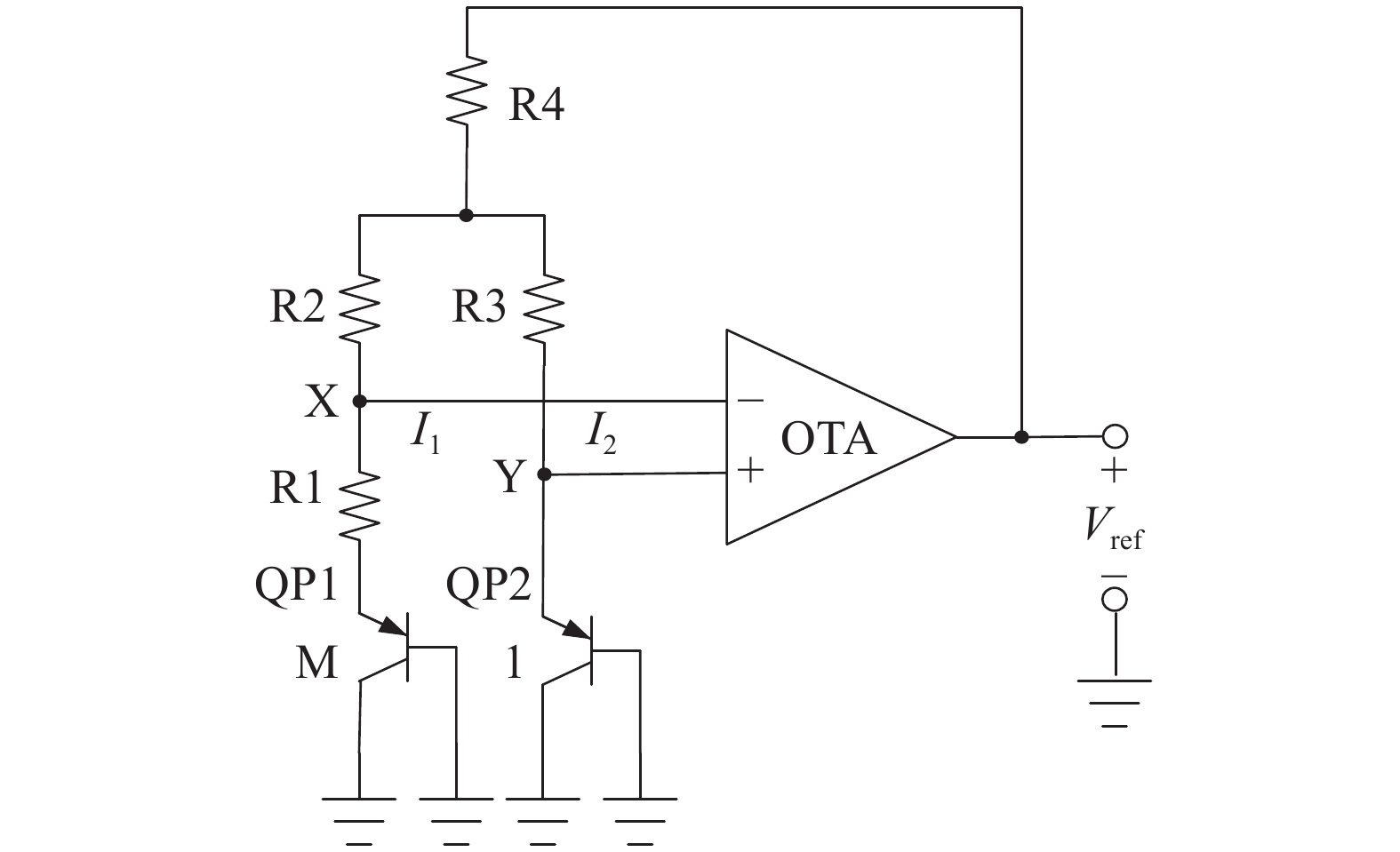Download: 图 1 传统带隙基准电路

 $V_X = V_Y$ (3)

 $I_1 = I_2 = \frac{{{V_{{\rm{be}}_2}} - {V_{{\rm{be_1}}}}}}{{R_1}} = \frac{{\Delta {V_{{\rm{be}}}}}}{{R_1}}$

 ${V_{{\rm{be}}}} = {V_T}\ln \frac{I}{{m{I_S}}}$
 $\Delta {V_{{\rm{be}}}} = {V_T}\ln m$

 $V_{\rm{ref}} = {V_{{\rm{be1}}}} + {V_T}\frac{{(R_1 + R_2 + 2R_4)}}{{R_1}}\ln m$ (7)

 $\frac{\partial V_{{\rm{ref}}}}{\partial T}=\frac{\partial V_{{\rm{b e}}_ 1}}{\partial T}+\frac{\partial V_{T}}{\partial T} \frac{\left(R_{1}+R_{2}+2 R_{4}\right)}{R_{1}} \ln m=0$ (8)

2 电路设计与实现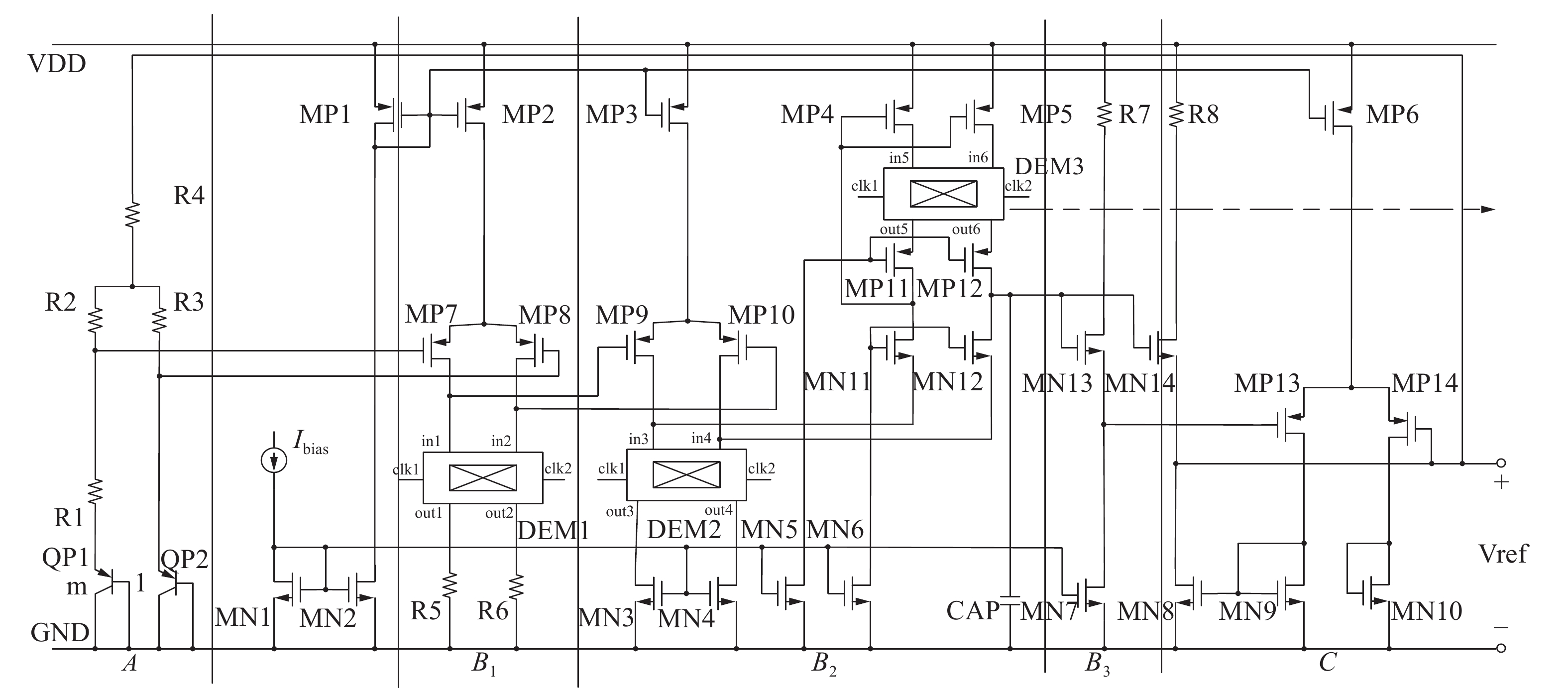Download: 图 2 本文提出的带隙基准电路
2.1 电路结构说明

 $V_{\rm{ref}} = {V_{{\rm{be_1}}}} + {V_T}\frac{{(R_1 + R_2 + 2R_4)}}{{R_1}}\ln m$

B部分为运算放大器，为两级放大，第一级B1为普通的双输入双输出的共源差分电路，其增益为：

 ${A_{{V_1}}} = {g_{{m_7}}}{R_5}$

 ${A_{{V_2}}} = {g_{{m_{12}}}}{r_{{o_{12}}}}{g_{{m_{10}}}}{r_{{o_{10}}}}$ (11)

B3为共漏结构的缓冲器，能够减少运算放大器的功耗以及噪声。

C部分为电压稳定电路，通过负反馈减少由于输入电压的变化带来对输出基准电压的影响。

B部分性能的好坏直接影响到式（3）能否成立，该部分性能指标是增益以及器件匹配程度，前面的分析已经得出该放大器具有大的增益，所以最大的影响来源于器件的匹配程度。

2.2 电路元件失配分析

1）B1中MP7与MP8、R5与R6的失配；

2）B2中MP9与MP10、MN3与MN4的失配；

3）B2中MP4、MP11组成的支路与MP5、MP12组成的支路的失配。

2.3 动态元件匹配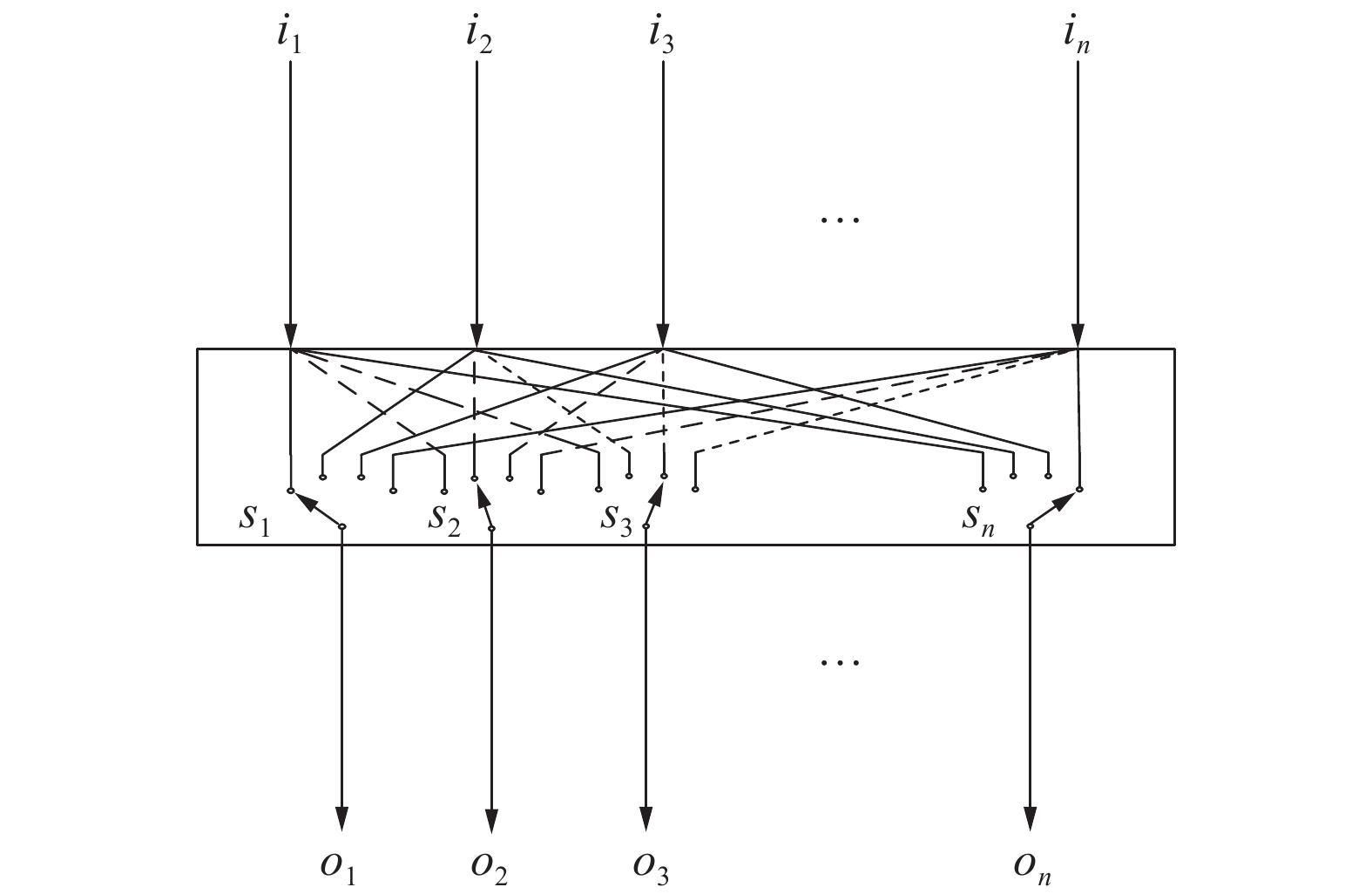Download: 图 3 动态元件匹配等效结构

 $\left[ \begin{gathered} {o_1} \\ {o_2} \\ \vdots \\ {o_n} \\ \end{gathered} \right] = \left[ {\begin{array}{*{20}{c}} {{p_{11}}}&{{p_{12}}}& \ldots &{{p_{1n}}} \\ {{p_{21}}}&{{p_{22}}}& \ldots &{{p_{2n}}} \\ \vdots & & & \vdots \\ {{p_{n1}}}&{{p_{n2}}}& \ldots &{{p_{nn}}} \end{array}} \right]\left[ \begin{gathered} {t_1}{i_1} \\ {t_2}{i_2} \\ \vdots \\ {t_n}{i_n} \\ \end{gathered} \right]$ (12)

 $\left[ \begin{gathered} {o_1} \\ {o_2} \\ \vdots \\ {o_n} \\ \end{gathered} \right] = \left[ {\begin{array}{*{20}{c}} {\dfrac{1}{n}}&{\dfrac{1}{n}}& \ldots &{\dfrac{1}{n}} \\ {\dfrac{1}{n}}&{\dfrac{1}{n}}& \ldots &{\dfrac{1}{n}} \\ \vdots & & & \vdots \\ {\dfrac{1}{n}}&{\dfrac{1}{n}}& \ldots &{\dfrac{1}{n}} \end{array}} \right]\left[ \begin{gathered} {t_1}{i_1} \\ {t_2}{i_2} \\ \vdots \\ {t_n}{i_n} \\ \end{gathered} \right]$ (13)

 ${o_i} = \frac{1}{n}\displaystyle\sum\limits_{j = 1}^n {{t_j}{i_j}}$

 $\left[ {\begin{array}{*{20}{c}} {{V_{{{o_1}}}}} \\ {{V_{{{o_2}}}}} \end{array}} \right] = \left[ {\begin{array}{*{20}{c}} {\dfrac{1}{2}}&{\dfrac{1}{2}} \\ {\dfrac{1}{2}}&{\dfrac{1}{2}} \end{array}} \right]\left[ \begin{gathered} {V_{i_1}}t \\ {V_{i_2}}t \\ \end{gathered} \right]$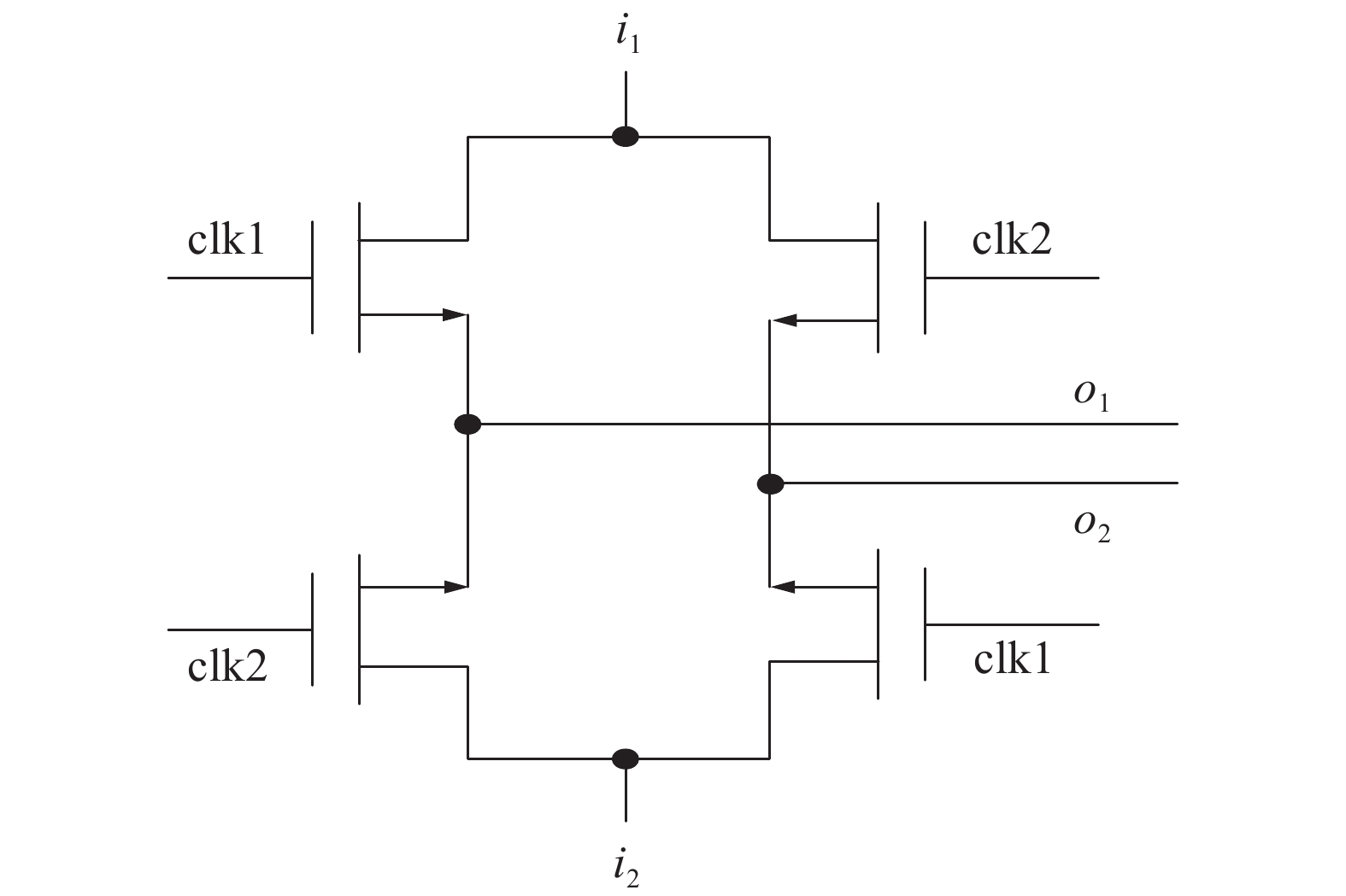Download: 图 4 动态元件匹配实现Download: 图 5 动态元件匹配时钟信号
3 仿真结果与分析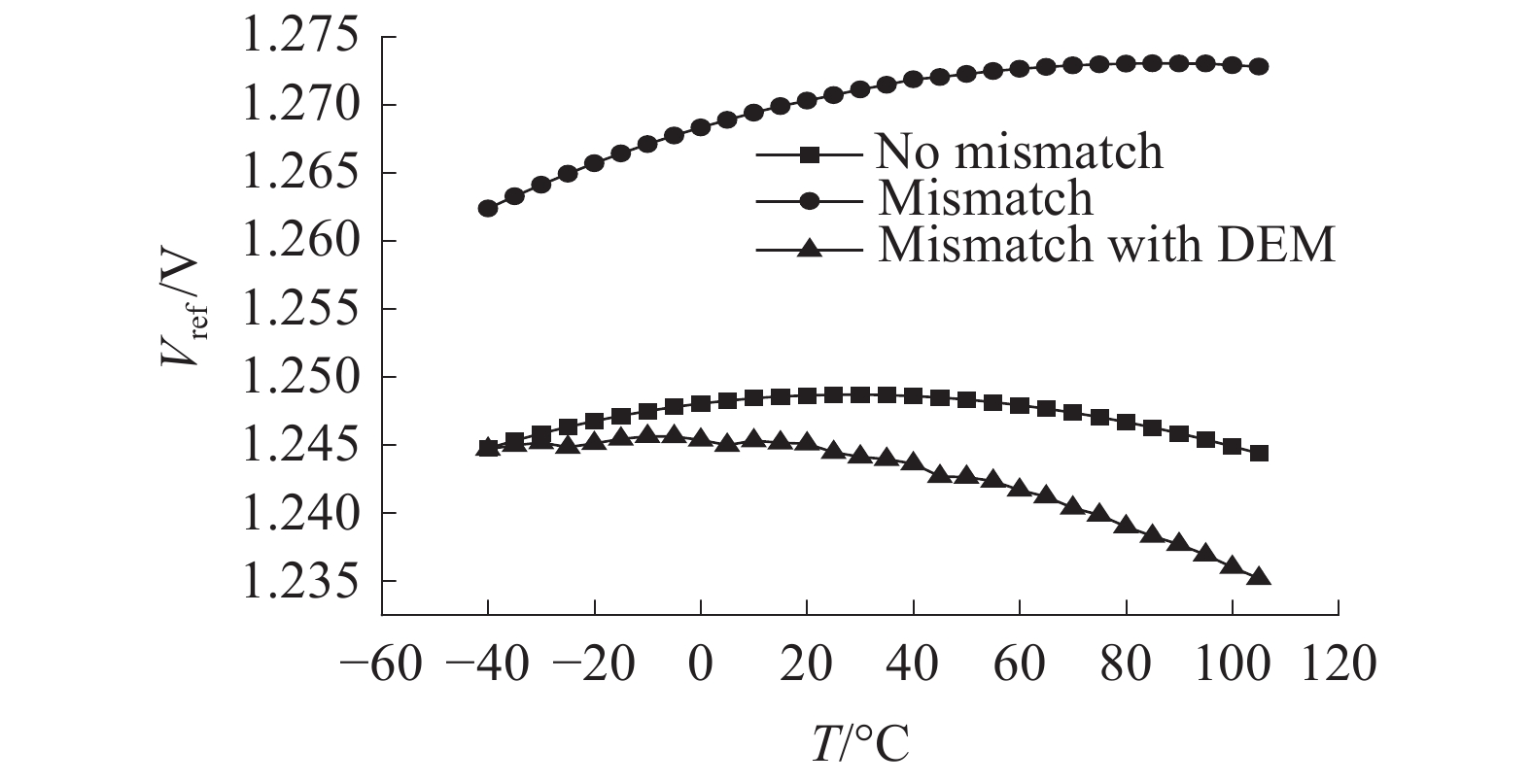Download: 图 6 温度特性仿真结果对比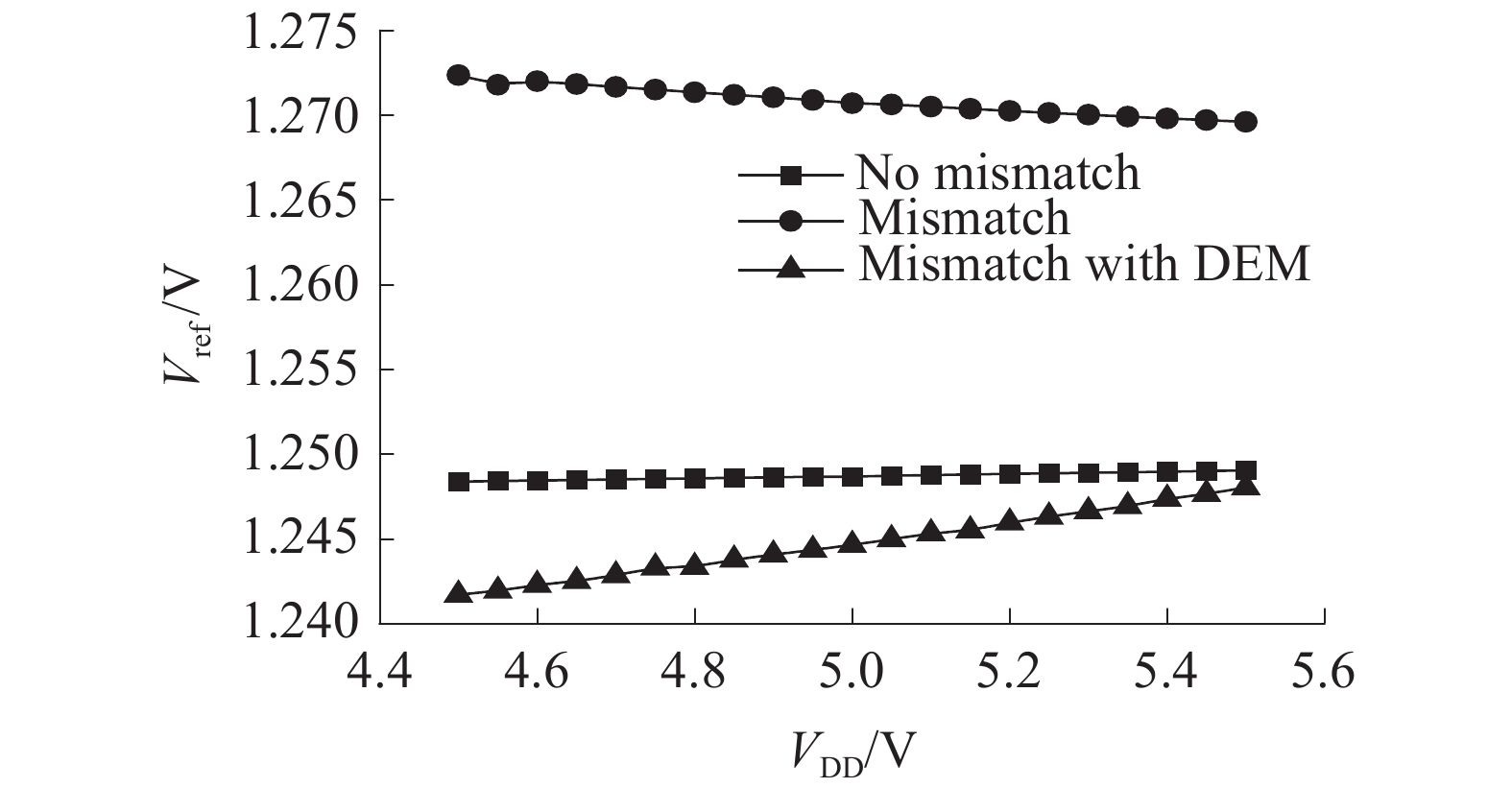Download: 图 7 电压特性仿真结果对比表 1 不同工艺角基准电压输出
4 结论

1）通过对电路的温度特性仿真表明，5.0 V供电、温度在233 K（−40 ℃）~378 K（105 ℃）变化时，相比于没有使用动态元件匹配的电路，该电路最少能够减小68%的误差；

2）通过对电路的电压特性仿真表明，25 ℃、电压在4.5~5.5 V变化时，相比于没有使用动态元件匹配的电路，该电路最少能够减小70%的误差；

3）在不同工艺角情况下，相比于没有使用动态元件匹配的电路，该电路最少能够减小68%的误差。

  RAZAVI B. Design of analog CMOS integrated circuits[M]. 2nd ed. New York: McGraw-Hill Education, 2017: 301–324. (0)  GRAY P R, HURST P J, LEWIS S H, et al. Analysis and design of analog integrated circuits[M]. 5th ed. New York: Wiley, 2009: 321–326. (0)  廖陆威, 冯全源. 无源标签中的全MOS电压基准源[J]. 应用科技, 2018, 45(3): 35-38, 43. (0)  秦少宏, 胡永贵, 胡云斌, 等. 一种高PSRR无电阻带隙基准源[J]. 微电子学, 2017, 47(4): 457-460. (0)  刘帘曦, 杨银堂, 朱樟明. 基于MOSFET失配分析的低压高精度CMOS带隙基准源[J]. 西安电子科技大学学报(自然科学版), 2005, 32(3): 348-352. DOI:10.3969/j.issn.1001-2400.2005.03.005 (0)  贺炜, 冯全源. 一种高温度性能的带隙基准源[J]. 电子器件, 2015, 38(1): 18-22. DOI:10.3969/j.issn.1005-9490.2015.01.005 (0)  曹璐, 刘宏, 田彤. 一种采用数字修调技术的低温漂带隙基准设计[J]. 电子设计工程, 2017, 25(5): 150-153, 157. (0)  佛朗哥·马洛贝蒂. 数据转换器[M]. 程军, 陈贵灿, 译. 西安: 西安交通大学出版社, 2013: 218. (0)  VAN DE PLASSCHE R. Dynamic element matching for high-accuracy monolithic D/A converters[C]//Proceedings of IEEE International Solid-state Circuits Conference Digest of Technical Papers. Philadelphia, PA, USA, 1976: 148–149. (0)  NYS O J A P, HENDERSON R K. An analysis of dynamic element matching techniques in sigma-delta modulation[C]//Proceedings of IEEE International Symposium on Circuits and Systems Circuits and Systems Connecting the World. Atlanta, GA, USA, 1996: 231–234. (0)  HENDERSON R, NYS O J A P. Dynamic element matching techniques with arbitrary noise shaping function[C]//IEEE International Symposium on Circuits and Systems Circuits and Systems Connecting the World. Atlanta, GA, USA, 1996: 293–296. (0)  杨晓春, 于奇, 宋文青, 等. 一种采用斩波调制的高精度带隙基准源的设计[J]. 微电子学与计算机, 2013, 30(1): 86-89. (0)